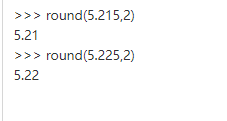• 1、Math.round() “四舍五入”，该函数返回的是一个四舍五入后的的整数 double d = 3.1415926; double d2 = 18.58; double d3 = -15.23; double d4 = -16.85; long round1 = Math.round(d); //...
1、Math.round()  “四舍五入”， 该函数返回的是一个四舍五入后的的整数

        double d = 3.1415926;
double d2 = 18.58;
double d3 = -15.23;
double d4 = -16.85;
long round1 = Math.round(d);    // 结果 3
long round2 = Math.round(d2);   // 结果 19
long round3 = Math.round(d3);   // 结果 -15
long round4 = Math.round(d4);   // 结果 -17

当小数点第一位=5是，这时要注意正负数的结果。例如

        double d5 = -16.5;
double d6 = 16.5;
long round5 = Math.round(d5);   // 结果 -16
long round6 = Math.round(d6);   // 结果 17

总结：负数，小数点第一位是5时，直接舍去，整数部分不 +1； 正数，小数点第一位是5时，往整数部分 +1

2、Math.ceil()  “向上取整”， 即小数部分直接舍去，并向正数部分进1

        double d = 3.1415926;
double d2 = 18.58;
double d3 = -15.23;
double d4 = -16.85;
double d5 = -16.5;
double d6 = 16.5;

double ceil1 = Math.ceil(d);    // 结果 4.0
double ceil2 = Math.ceil(d2);   // 结果 19.0
double ceil3 = Math.ceil(d3);   // 结果 -15.0
double ceil4 = Math.ceil(d4);   // 结果 -16.0
double ceil5 = Math.ceil(d5);   // 结果 -16.0
double ceil6 = Math.ceil(d6);   // 结果 17.0

【注】该数为小数时，小数部分直接舍去

3、Math.floor()  “向下取整” ，即小数部分直接舍去

        double d = 3.1415926;
double d2 = 18.58;
double d3 = -15.23;
double d4 = -16.85;
double d5 = -16.5;
double d6 = 16.5;

double floor1 = Math.floor(d);    // 结果 3.0
double floor2 = Math.floor(d2);   // 结果 18.0
double floor3 = Math.floor(d3);   // 结果 -16.0
double floor4 = Math.floor(d4);   // 结果 -17.0
double floor5 = Math.floor(d5);   // 结果 -17.0
double floor6 = Math.floor(d6);   // 结果 16.0

【注】 Math.floor()容易出现精度问题，举个最简单例子:

对小数 8.54 保留两位小数(虽然它已经保留了 2 位小数)：

Math.floor(8.54*100)/100 // 输出结果为 8.53, 注意是 8.53 而不是 8.54。

所以这种函数慎用。


展开全文Math
• ## round()

千次阅读 2019-01-13 17:04:00
round()用于对一个数值进行四舍五入，如果接收两个参数，则第二个参数表示保留多少位小数 In : round(1.5324) Out: 2.0 In : round(1.5324, 2) Out: 1.53 转载于:...
round() 用于对一个数值进行四舍五入，如果接收两个参数，则第二个参数表示保留多少位小数

In : round(1.5324)
Out: 2.0

In : round(1.5324, 2)
Out: 1.53

转载于:https://www.cnblogs.com/pzk7788/p/10263281.html
展开全文• ## 详解python中的round()函数

万次阅读 多人点赞 2019-04-06 17:46:36
round()是python自带的一个函数，用于数字的四舍五入。 但是round()的输出结果与Python的版本有关： 在python2中，round(1.0/2.0)=0；在python3中，round(1.0/2.0)=1 $python Python 2.7.8 (default, Jun 18 ... round()是python自带的一个函数，用于数字的四舍五入。 但是round()的输出结果与Python的版本有关： 在python3中，round(1.0/2.0)=0；在python2中，round(1.0/2.0)=1 $ python
Python 2.7.8 (default, Jun 18 2015, 18:54:19)
[GCC 4.9.1] on linux2
>>> round(0.5)
1.0

\$ python3
Python 3.4.3 (default, Oct 14 2015, 20:28:29)
[GCC 4.8.4] on linux
>>> round(0.5)
0



使用方法：round(number,digits)

digits>0，四舍五入到指定的小数位
digits=0, 四舍五入到最接近的整数
digits<0 ，在小数点左侧进行四舍五入
如果round()函数只有number这个参数，等同于digits=0
四舍五入规则：

要求保留位数的后一位<=4，则舍去3，如5.214保留小数点后两位，结果是5.21
要求保留位数的后一位“=5”，且该位数后面没有数字，则不进位，如5.215，结果为5.21
要求保留位数的最后一位“=5”，且该位数后面有数字，则进位，如5.2151，结果为5.22
要求保留位数的最后一位“>=6”，则进位。如5.216，结果为5.22
例子：展开全文• 菜鸟教程中介绍到，round() 函数作用就是，返回浮点数x的四舍五入值。 round( x [, n] ) 参数x，n均为数值表达式，返回值为x的四舍五入值。n为保留的小数位数，不加n则只保留x四舍五入后的整数部分。 &amp;amp...
round( )函数简介
菜鸟教程中介绍到，round() 函数作用就是，返回浮点数x的四舍五入值。
> round( x [, n]  )

参数x，n均为数值表达式，返回值为x的四舍五入值。n为保留的小数位数，不加n则只保留x四舍五入后的整数部分。
>>> round(2.3)
2
>>> round(2.45, 1)
2.5

特殊情况
上面的结果并没有错误，这里再用2.675测试一下：
>>> round(2.675, 2)
2.67

显然结果不符合四舍五入的规则。为什么会这样呢？原因是：round()函数只有一个参数，不指定位数的时候，返回一个整数，而且是最靠近的整数，类似于四舍五入，当指定取舍的小数点位数的时候，一般情况也是使用四舍五入的规则，但是碰到.5的情况时，如果要取舍的位数前的小数是奇数，则直接舍弃，如果是偶数则向上取舍。
这也就解释了上述现象。可这样一来用round()函数取浮点数的四舍五入值不就变得不可靠了嘛？这样的函数设计的意义何在？网上搜了一圈答案，觉得这个说法比较准确：
python3（注意python2 和 3的round()是不一样的，这里仅以python3作说明）中round()对浮点数的取舍遵循的是“四舍六入五平分”，“五平分”就是根据取舍的位数前的小数奇偶性来判断，奇偶平分，符合公平性原则（四舍五入不是公平的），这样一来也就保证了在数据量较大的情况下，筛选数据的真实性。（数学渣，不知道这样理解对否……）
为什么需要平分呢？原因就是部分小数无法用二进制完整表示，如1.15，转为二进制将是很长的一串数字：1.0010011001100110011001100110011001100110011001100110011   这可不是简单的几个字节就能存放下的。因此这里就出现了取舍的问题。
那么正确的四舍五入是否无法实现了呢？当然是有解决办法的。比如，当你需要四舍五入保留两位小数的时候，可以将数值乘以100再除以100.0：
>>> round(2.675 * 100)/100.0
2.68

这样可以解决部分浮点数四舍五入的问题。为什么是部分呢？笔者发现：
>>> round(2.135*100)/100.0
2.13检验下过程：
>>> 2.135*100
213.49999999999997

WTF！是精度问题嘛！
>>> Decimal(2.135)*100
Decimal('213.4999999999999786837179272')无法理解是不是！！二进制的世界正常人真的不懂，盼望一下未来有可以直接计算十进制的硬件诞生吧。
总结
在用round()函数进行四舍五入时，如果你对结果有十足把握，并且这就是你想要的结果，那就放心大胆地使用。不然就老老实实写个函数来实现吧，这不是什么难事。


展开全文python 数值计算
• ## Java中Math.round()的取整规则

万次阅读 多人点赞 2017-11-01 14:25:54
做Java的面试题时遇到了以下这题，百度了一下Math.round()的修约规则，有的说是四舍五入，有的说是四舍六入，发现和我学分析化学时用的数字修约规则（四舍六入五成双）很像，所以验证一下； 原题：Math.round(11.5)...java 面试题
• trunc()、ceiling()、floor()、round()函数在R语言中都是取整，但是用法上有一定的区别。 函数 用法 trunc() 去除小数部分 ceiling() 向上取整 floor() 向下取整 round() 一般情况下四舍五入，但会受...r语言
• MySql AVG() ROUND()函数 AVG()函数： 作用： 平均值计算 用法： 1、计算一组数据的平均值 例：查询学生成绩表中各科成绩平均值 SELECT subject,ROUND(AVG(score),2) as score_avg FROM study_score GROUP BY ...mysql
• Math.round() “四舍五入”， double d = 3.1415926; double d2 = 18.58; double d3 = -15.23; double d4 = -16.85; long round1 = Math.round(d); // 结果 3 long round2 = Math.round(d2); // 结果 19 long...
• iOS开发中我们常用的函数abs() floor() ceil() round() fmax() fmin() fmod()  NSLog(@"abs %i",abs(-3));     NSLog(@"abs %d",abs(-4));     //floor() 向下取整   NSLog(@"floorf...iOS
• 首先知道floor()、ceil()和round()函数都在math.h头文件中。 三个与取整有关的方法：ceil,floor,round,这些方法的作用于它们的英文名称的含义相对应： floor()的英文含义是地板，所以是向下取整，方便记忆叫它地板...
• ceil() 向上取整 floor() 向下取整 round() 四舍五入
• Math.round() 就是数学中的四舍五入，举例： System.out.println("Math.round(1.2)="+Math.round(1.2)); System.out.println("Math.round(1.5)="+Math.round(1.5)); System.out.println("Math.round(1.7)="+Math....java
• round（）函数 round() 方法返回浮点数x的四舍五入值。 语法 以下是 round() 方法的语法: round( x [, n] ) 参数 x -- 数值表达式。 n -- 数值表达式。 返回值 返回浮点数x的四舍五入值。 实例 以下展示...
• 四、round() 五、divmod() 一、min() min()函数：括号内带数字集合，求集合的最小值 # 将括号内的数据集合可以是列表，也可以直接元组 二、max() max() 函数：括号内带数字集合，求集合的最大值 # 将括号...
• ## 在python中round()方法的作用

万次阅读 多人点赞 2019-03-19 22:52:21
** python中round()方法的作用** ** round()方法的作用是对于浮点数进行四舍五入。 round(x) 返回浮点数x的四舍五入的值 &gt;&gt;&gt; round(2.6) 3 &gt;&gt;&gt; round(2.5) 2 ...
• select round(2.6); OK _c0 3.0 Time taken: 1.331 seconds, Fetched: 1 row(s) hive (default)> SELECT floor(2.6); OK _c0 2 Time taken: 0.094 seconds, Fetched: 1 row(s) hive (default...hive 函数
• Math.round() 就是数学中的四舍五入，举例： System.out.println("Math.round(1.2)="+Math.round(1.2)); System.out.println("Math.round(1.5)="+Math.round(1.5)); System.out.println("Math.round(1.7)="+...java
• Python3 math.ceil()、math.floor()、//、int()、round()取整问题 1. 向上取整：math.ceil()，往较大的方向取 import math math.ceil(2.1) 3 math.ceil(2.8) 3 math.ceil(-2.1) -2 math.ceil(-2.9) -2 2. ...int()
• 1.Random()、Math.random()、Math.round()函数的区别;1.Random()、Math.random()、Math.round()函数的区别;1.Random()、Math.random()、Math.round()函数的区别;1）Random()：创建一个新的随机数生成器。此构造方法...
• Math.round()为四舍五入，但是负数则会舍弃-5。 System.out.println(Math.round(2.6));//3 System.out.println(Math.round(-1.6));//-2 System.out.println(Math.round(-1.5));//-1 Math.floor()为直接去掉小数保留...
• ## Matlab中的round()函数

万次阅读 多人点赞 2019-08-14 14:12:46
语法形式只有1种：Y = round(X)，这里的X可以是数，向量，矩阵，输出对应。 栗子1： 正数时： round(2.1) = 2; round(2.5) = 3; round(2.8) = 3 负数时： round(-2.1) = -2; round(-2.5) = -3; round(-2.8) = -3 ...Matlab
• 刷题看到Math.round()题目，就知道又到了翻文档的时候了 返回最接近参数的 long。结果将舍入为整数：加上 1/2，对结果调用 floor 并将所得结果强制转换为 long 类型。换句话说，结果等于以下表达式的值： (long)...
• ## Java Math.round()

千次阅读 2019-09-20 19:42:47
标题关于java的Math.round()函数 round 是 4 舍 5 入的计算，入的时候是到大于它的整数。 round 表示”四舍五入”，算法为Math.floor(x+0.5) ，即将原来的数字加上 0.5 后再向下取整，所以 Math.round(11.5) 的结果...java
• c语言 round()函数的使用 round(double); 可以认为是一个四舍五入的函数，返回距离参数最近的整数，如果参数值正好在两个整数的中间位置，则返回距离0较远的那一个整数（即正数则返回大于参数的整数，负数则返回小于...c语言
• Tags:JavaScriptMath ceil()、floor()、round()方法Math.ceil()功能：对一个数进行上取整。 语法：Math.ceil(x) 参数： x：一个数值。 返回值：返回大于或等于x，并且与之最接近的整数。 注：如果x是正数，则把...math javascript
• 小数经常需要取整，在js给我们提供了三个舍入函数：round()， ceil() ，floor()。他们各自有各自的用途，在日常生活会和科学研究的数据中，经常就要进行四舍五入，这也是我们从小到大遇到最多的舍入取整方式，js的......